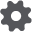# Hysteretic Spring

The Hysteretic Spring contact model allows plastic deformation behaviors to be included in the contact mechanics equations, resulting in particles behaving in an elastic manner up to a predefined stress. Once this stress is exceeded, the particles behave as though undergoing plastic deformation. The result is that large overlaps are achievable without excessive forces acting upon them, thus representing a compressible material. Hysteretic Spring normal force calculation is based on the Walton-Braun theory described in the following references (Walton and Braun 1986), (Walton and Braun 1986).In particular, the normal force, FN, is given by:where K1 and K2 are the loading and unloading stiffness respectively, δn, is normal overlap and δ0 is the residual overlap. Loading stiffness, K1, is related to the yield strength of each material participating in contact, Y1 and Y2, as follows (Walton O., 2006):Here R* is the equivalent radius of the two particles participating in the contact. The following expression for coefficient of restitution:allows for the determination of K>2 from K1 (Walton O. , 2006). Residual overlap is updated every time step according to the following rule:The main energy dissipation mechanism is due to the difference in spring stiffness between loading and unloading phases. The need for an additional velocity dependent dissipation mechanism, to suppress possible undamped low amplitude oscillations, was suggested in (Walton O. , 2006). In EDEM's Hysteretic Spring model, this mechanism was implemented in a way similar to the linear spring normal damping force scaled by a dimensionless user set parameter ‘Damping Factor’, bn, as follows:Here k is either K1 or K2. The rest of the variables and factors in the above expression are explained in the Linear Spring contact model section.

Since EDEM's Hysteretic Spring model for the normal force can be considered as an extension of linear spring model for normal force it makes sense to use tangential components of linear spring model for tangential forces. In particular, more general form of linear spring elastic tangential force is used in Hysteretic Spring model in which the force depends on user set stiffness factor, γt, as follows:Unlike for the normal component, the tangential damping force is not scaled by a damping factor:For Particles, a default value for the Yield Strength is estimated from the Young’s Modulus E and Radius of the particle R according to the algorithm suggested in (Walton O. , 2006). First, the possible default value is calculated from:If this value is less than 100Pa it is replaced with a value of 0.003 * E.

For geometries, the default value is set to 1e+09 Pa.

 Interaction Configurable Parameters Position Particle to Particle, Particle to Geometry Click + to add particle-particle or particle-geometry interactions. Set the following interaction parameters:   Damping factor: this dimensionless parameter controls the amount of velocity dependent damping. Without this additional damping, small vibrations of particles can persist for a long time. Setting this parameter to zero suppresses only the velocity-dependent damping without affecting the main energy loss mechanism due to the nature of the linearized elasto-plastic model. The recommended value for this parameter is zero when modeling “aggressive” compression of materials and small non-zero value for small stain compression regimes.   Stiffness factor: This dimensionless parameter is defined as the ratio of tangential stiffness to normal loading stiffness and used in calculation of tangential forces at the contact. According to the literature, the typical value of this parameter is in the range between 0.7 and 1.   Also set the yield strength in units of Pa for each particle or geometry that has an interaction. EDEM offers a reasonable default value for this parameter estimated from material Shear Modulus. The user can, however, overwrite this default value. Last

Use:

1. Select the required category from the Interaction pulldown in the Physics section of the Creator.
2. Click the + drop-down list then select Hysteretic Spring.
3. Click the configuration buttonto define contact model parameters.